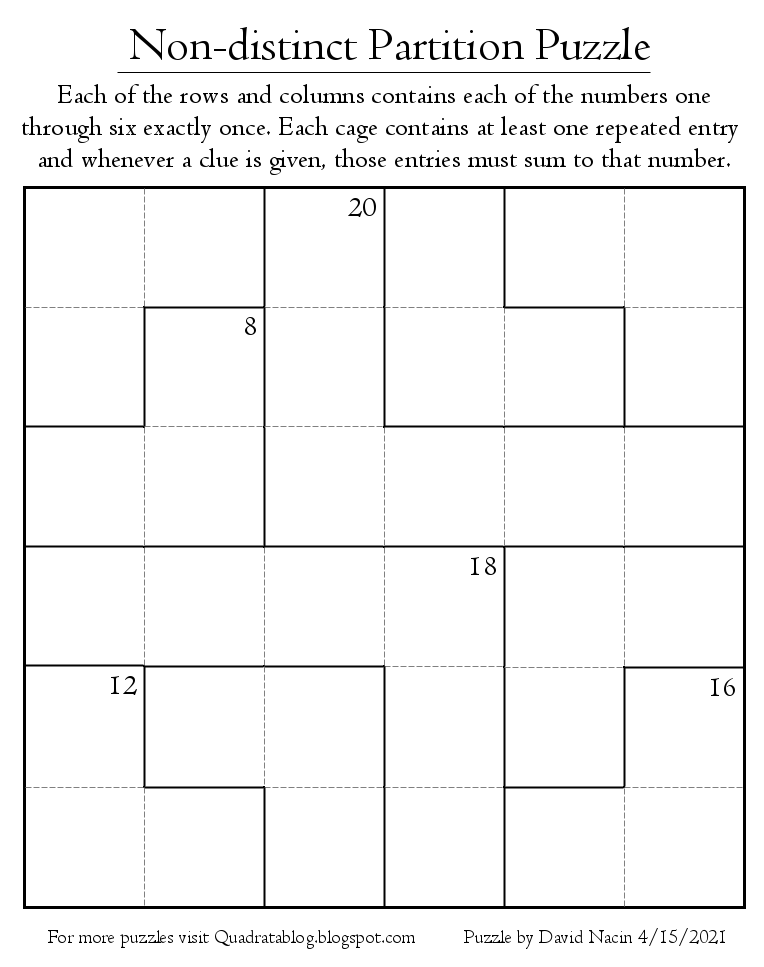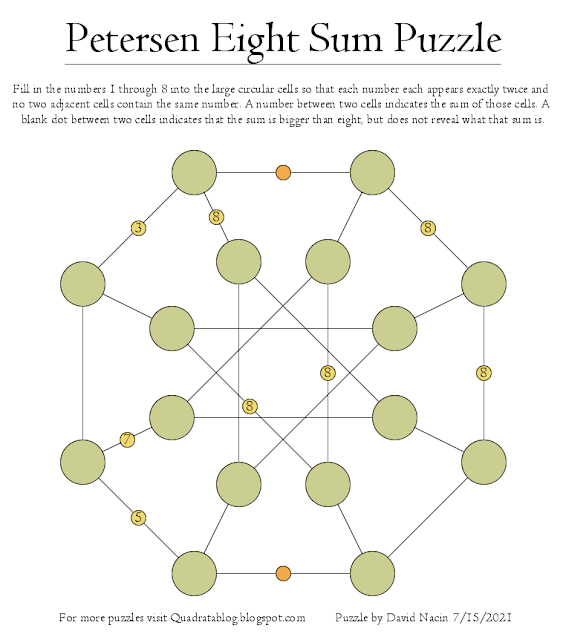## Quadrata: A Free Puzzle Blog

Links to puzzle sites all around the web
Posts: 19
Joined: Wed 12 Aug, 2020 6:20 pm

### Quadrata: A Free Puzzle Blog

Just wanted to let everyone here know about a puzzle website that I maintain. I post new original puzzle varieties there about twice a month. Today I posted three Sudoku variants where the clues are the greatest common divisors of the numbers in adjacent cells. This puzzle is below, and the solution and more info can be found at the blog at:Posts: 19
Joined: Wed 12 Aug, 2020 6:20 pm

### Re: Quadrata: A Free Puzzle Blog

Here are some small puzzles based around divisibility, with arrows revealing which number divides which. Dots indicate that neither divides the other. The first puzzle is solved to give you a visual instruction. The next three puzzles increase with difficulty as they go.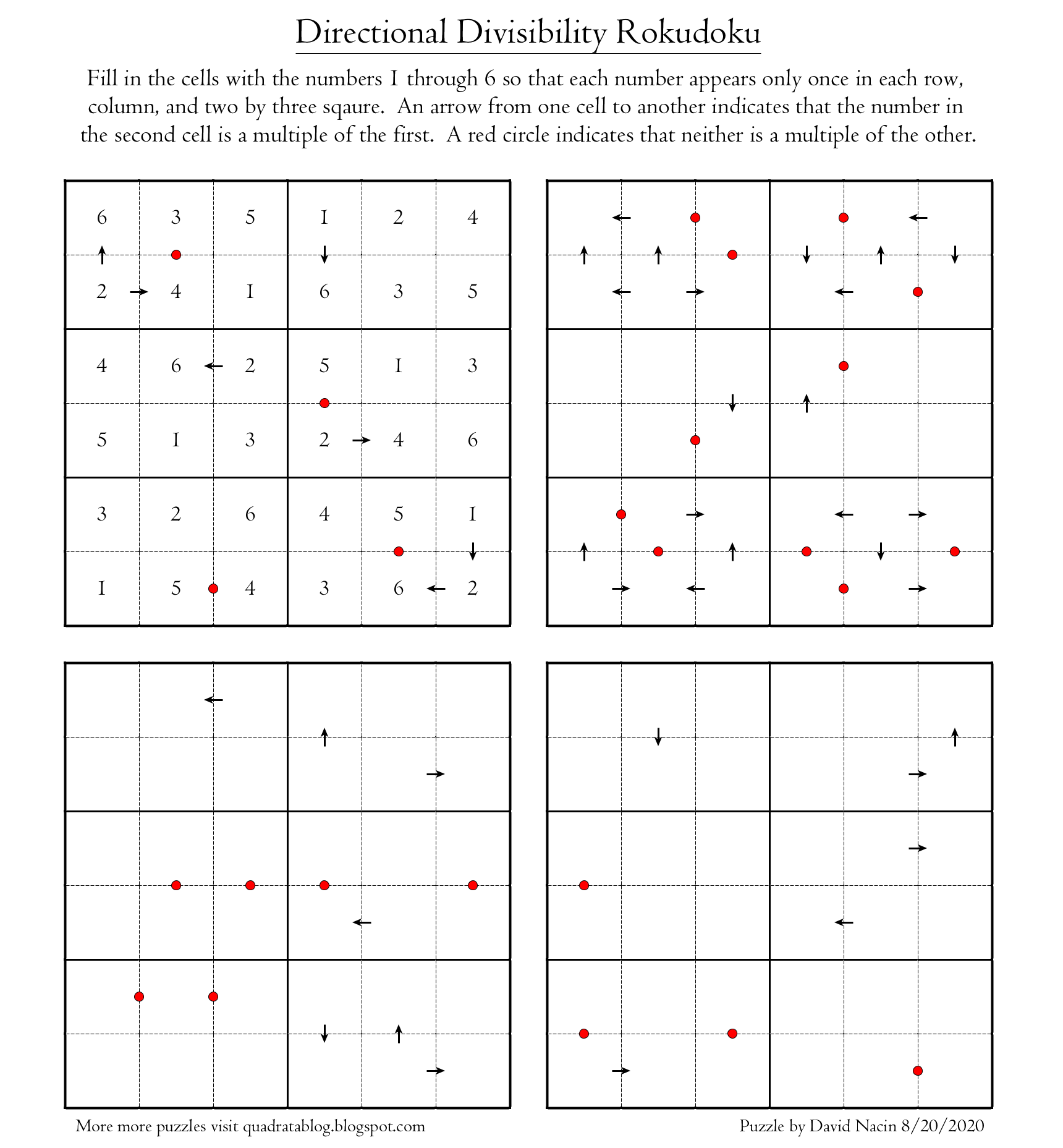Posts: 19
Joined: Wed 12 Aug, 2020 6:20 pm

### Re: Quadrata: A Free Puzzle Blog

Here's a 9x9 Sudoku where the clues tell you what the sum is of the two adjacent cells. The catch is that the numbers are only given when they are even. Thus a numberless dot reveals that the sum is odd without telling you what that sum is.Solution, a full PDF and more can be found at: https://quadratablog.blogspot.com/2020/ ... udoku.html

Posts: 19
Joined: Wed 12 Aug, 2020 6:20 pm

### Re: Quadrata: A Free Puzzle Blog

Here are some smaller 6x6 versions of my last puzzle, the even sum Sudoku. They start easy and get harder as they go. Rules are on the puzzle and the solution and PDF can be found at my blog here:Posts: 19
Joined: Wed 12 Aug, 2020 6:20 pm

### Re: Quadrata: A Free Puzzle Blog

Here's a Sudoku with clues based around the products of numbers in adjacent cells. Even products are fully revealed but odd products result in a numberless dot.

Solution and more at: https://quadratablog.blogspot.com/2020/ ... udoku.htmlPosts: 19
Joined: Wed 12 Aug, 2020 6:20 pm

### Re: Quadrata: A Free Puzzle Blog

Here's a puzzle I designed for a math conference that took place last weekend. You don't need to know much more than which numbers divide 2020 to attempt it, so I figured I would share it here as well. For more details and a solution visit my blog here: https://quadratablog.blogspot.com/2020/ ... uzzle.html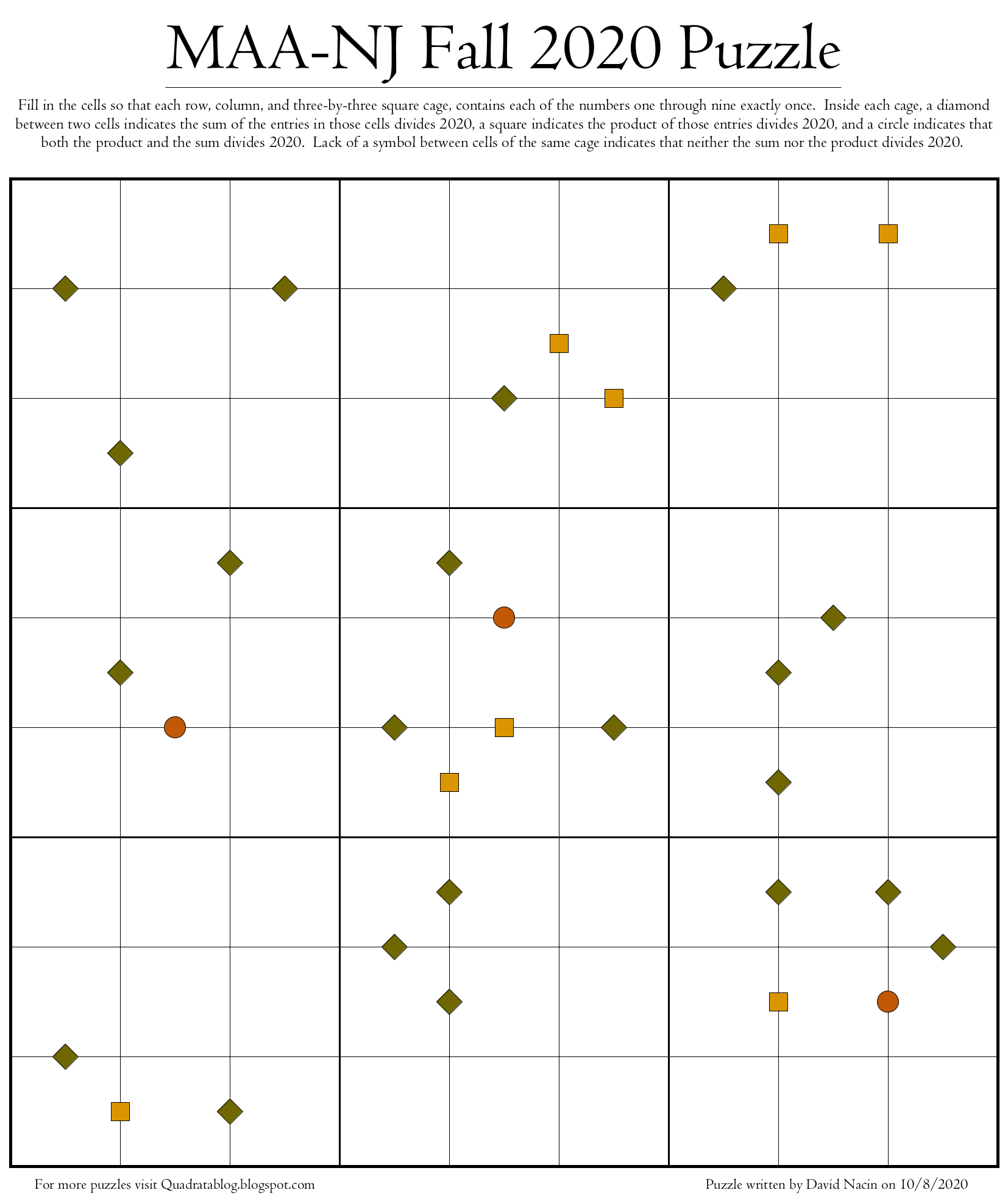Posts: 19
Joined: Wed 12 Aug, 2020 6:20 pm

### Re: Quadrata: A Free Puzzle Blog

This next puzzle is a little mathy in the sense that it uses the primes, but really all you need to know is that the first three prime numbers are 2, 3, and 5. Basically, if you see a number between cells then exactly one of those cells contains a prime, and that number tells you the sum. A diamond means that either neither of the numbers or both of the numbers must be prime. Other than that, the rules are identical to normal Sudoku with each number appearing exactly once in every row, column, and two-by-three cage.

For a solution, PDF, and zoomed in individual puzzles visit Quadrata at www.quadratablog.blogspot.com.Posts: 19
Joined: Wed 12 Aug, 2020 6:20 pm

### Re: Quadrata: A Free Puzzle Blog

Here's a larger version of my last puzzle, now in full 9x9 glory! If you’re new to prime numbers you only need to know that the first seven primes are 2, 3, 5, 7, 11, 13, and 17 to try it.

The numbers in this puzzle reveal the sum of the entries in adjacent cells only when exactly one of those numbers is prime. The diamonds reveal that either both or neither number is prime.Posts: 19
Joined: Wed 12 Aug, 2020 6:20 pm

### Re: Quadrata: A Free Puzzle Blog

Another new concept: Dots between cells indicate consecutive numbers, and the colors indicate the size. Dots are also placed whenever possible between cells of the same cage, so lack of a dot means something!Posts: 19
Joined: Wed 12 Aug, 2020 6:20 pm

### Re: Quadrata: A Free Puzzle Blog

I just made a snowflake puzzle for the holiday this year.

Consecutive numbers are numbers which are one apart such as 1 and 2, or 4 and 5. A lettered dot between diamonds means the numbers in those diamonds are consecutive, and a blank dot means they are not. In addition, the letter is ‘L’ for low if both are below 3, 'H’ for high if they both are above 3, and ’M’ otherwise. Remember to use each number six times and that adjacent diamonds never contain the same number.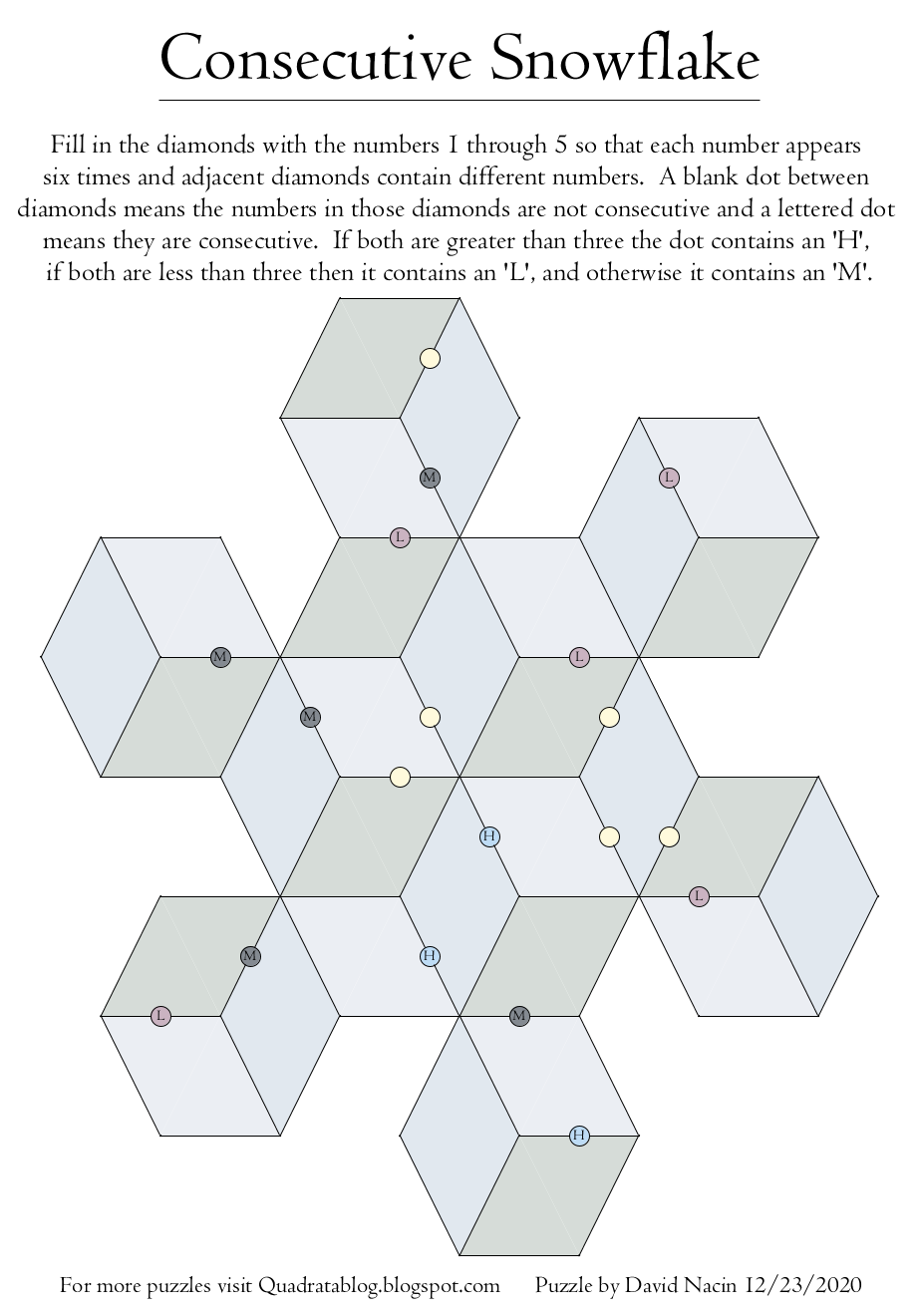Posts: 19
Joined: Wed 12 Aug, 2020 6:20 pm

### Re: Quadrata: A Free Puzzle Blog

With the success of the Queen’s Gambit, I’ve been getting requests for chess puzzles. I figured it was time to post a Queen’s Sudoku. Each row, column, and three-by-three cage must contain exactly three Queens. Some Queens are given, and the clues attached reveal the number of other pieces they can attack. Assume that pieces do not block other pieces from attack for purposes of that calculation.

For the solution visit: https://quadratablog.blogspot.com/2021/ ... udoku.html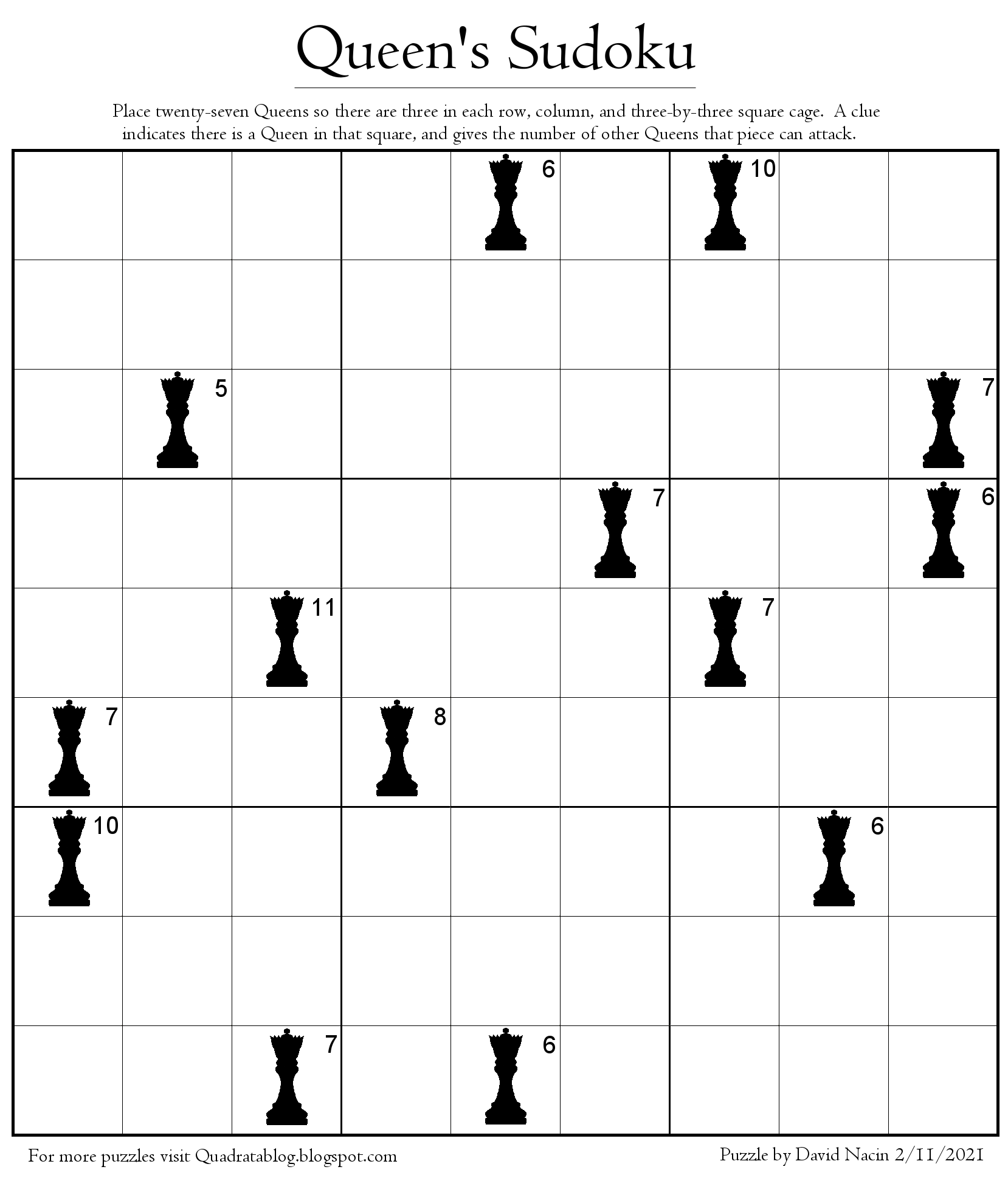Posts: 19
Joined: Wed 12 Aug, 2020 6:20 pm

### Re: Quadrata: A Free Puzzle Blog

Here's a 'word' puzzle I made where the clues represent the distance between letters in the alphabet. It's also a Sudoku in the sense that each symbol appears the same number of times in each row, column, and cage, even though the cages are now bendy and some symbols are repeated.

Clues are given whenever the letters are close to each other, specifically distance 3 or less. More info and solution at: https://quadratablog.blogspot.com/2020/ ... drata.html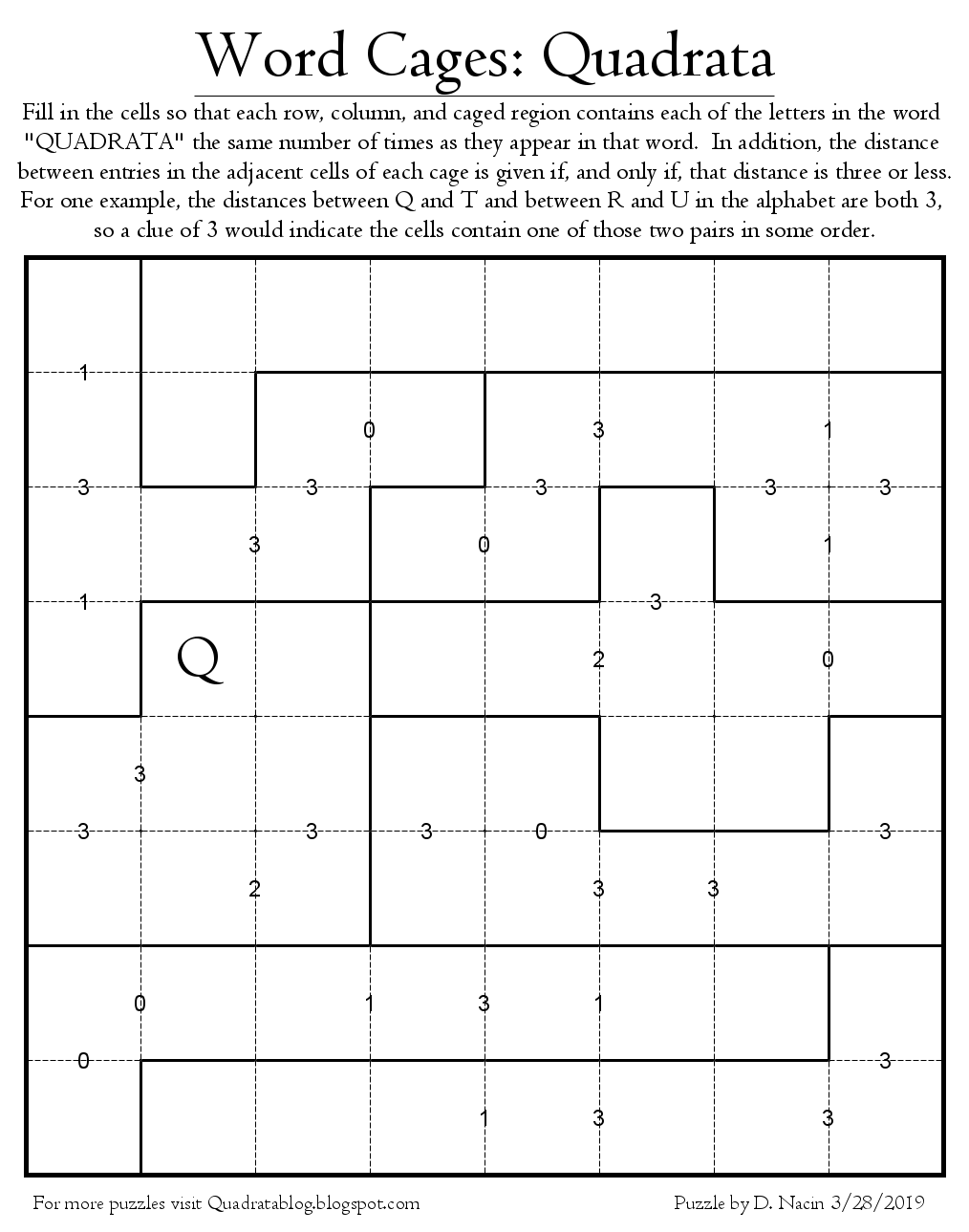Posts: 19
Joined: Wed 12 Aug, 2020 6:20 pm

### Re: Quadrata: A Free Puzzle Blog

The clues in this new style of puzzle depend on the number of primes in the two cells. A numeric clue indicates that exactly one of those numbers is prime and reveals the difference of the two numbers. A numberless clue indicates that either both or neither of those two entries are prime numbers. (Remember that 1 is not a prime!)Posts: 19
Joined: Wed 12 Aug, 2020 6:20 pm

### Re: Quadrata: A Free Puzzle Blog

Here's a new style of puzzle I made for Spring. Arrows point from larger numbers to smaller numbers. A dashed line means the numbers are not far apart (the difference is 1 or 2) and a solid line means the numbers are farther apart (the difference is 3 or 4.) Use each of the numbers 1 through 5 exactly three times without placing any number next to itself.Posts: 19
Joined: Wed 12 Aug, 2020 6:20 pm

### Re: Quadrata: A Free Puzzle Blog

New concept: Place 1 through 6 in the cells so each appears once in every row and column. The entries in each cage must be DISTINCT and sum to the clues when one is given.Posts: 19
Joined: Wed 12 Aug, 2020 6:20 pm

### Re: Quadrata: A Free Puzzle Blog

Here are some small easy versions of a larger puzzle I like to make. Each row and column contains all the numbers 1 through 4, no cage contains duplicate numbers, and the numbers in a cage sum to a clue whenever a clue is given.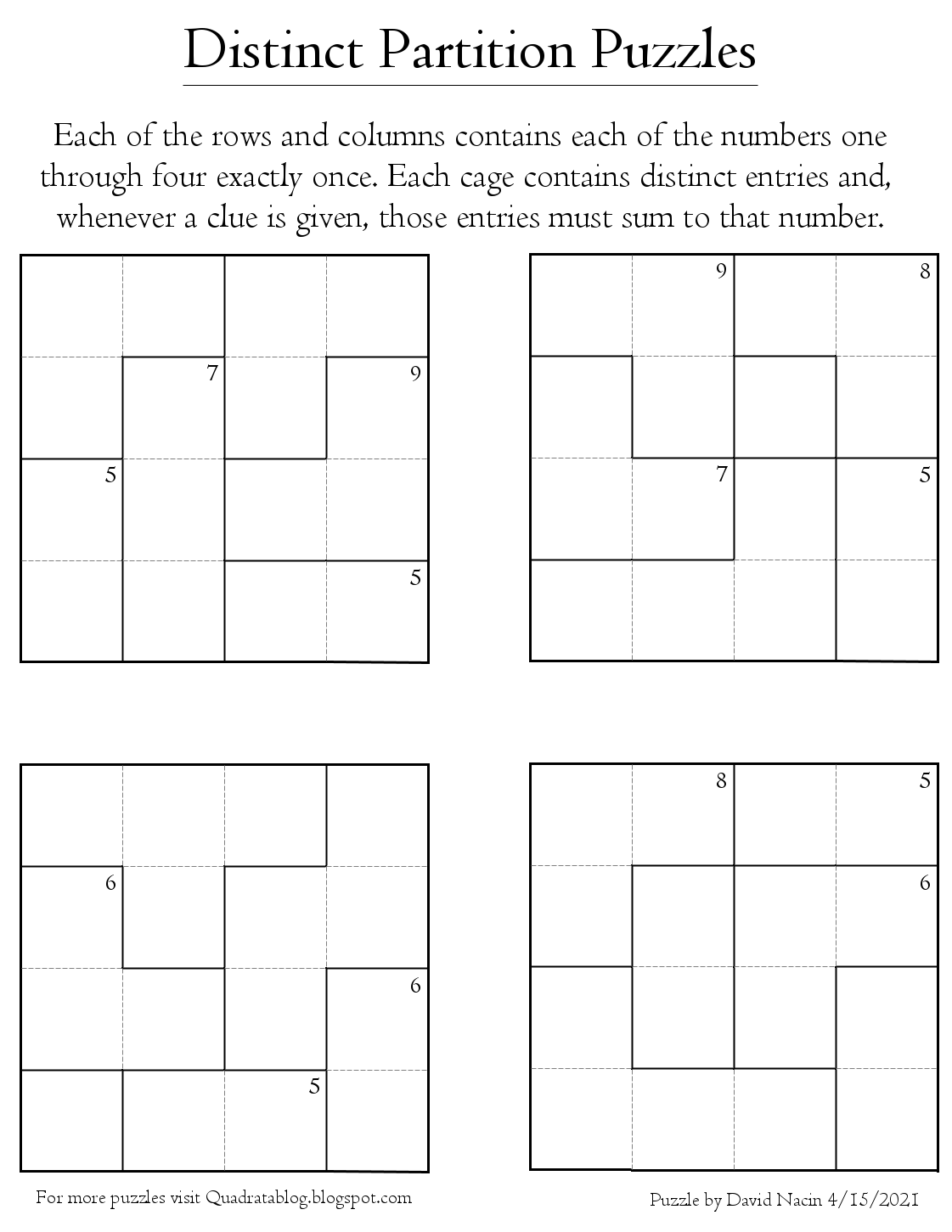Posts: 19
Joined: Wed 12 Aug, 2020 6:20 pm

### Re: Quadrata: A Free Puzzle Blog

Here's another calcudoku/KenKen style of puzzle but with a different twist. What if the entries in each cage are -forbidden- from being distinct? For example, a cage with three entries which add to 9 could be 4,4,1 but couldn't be 2,3,4, since those numbers are all different. Here are some small quick puzzles just to demonstrate the idea. All of the clues reveal the sums of the entries in that cage, but regardless of whether or not there is a clue, the entries in each cage cannot be distinct!Posts: 19
Joined: Wed 12 Aug, 2020 6:20 pm

### Re: Quadrata: A Free Puzzle Blog

In this puzzle, each row and each column contains the numbers 1 through 6 exactly once, but NO cage is allowed to contain all distinct numbers! Clues when given must equal the sum of the entries in that cage.